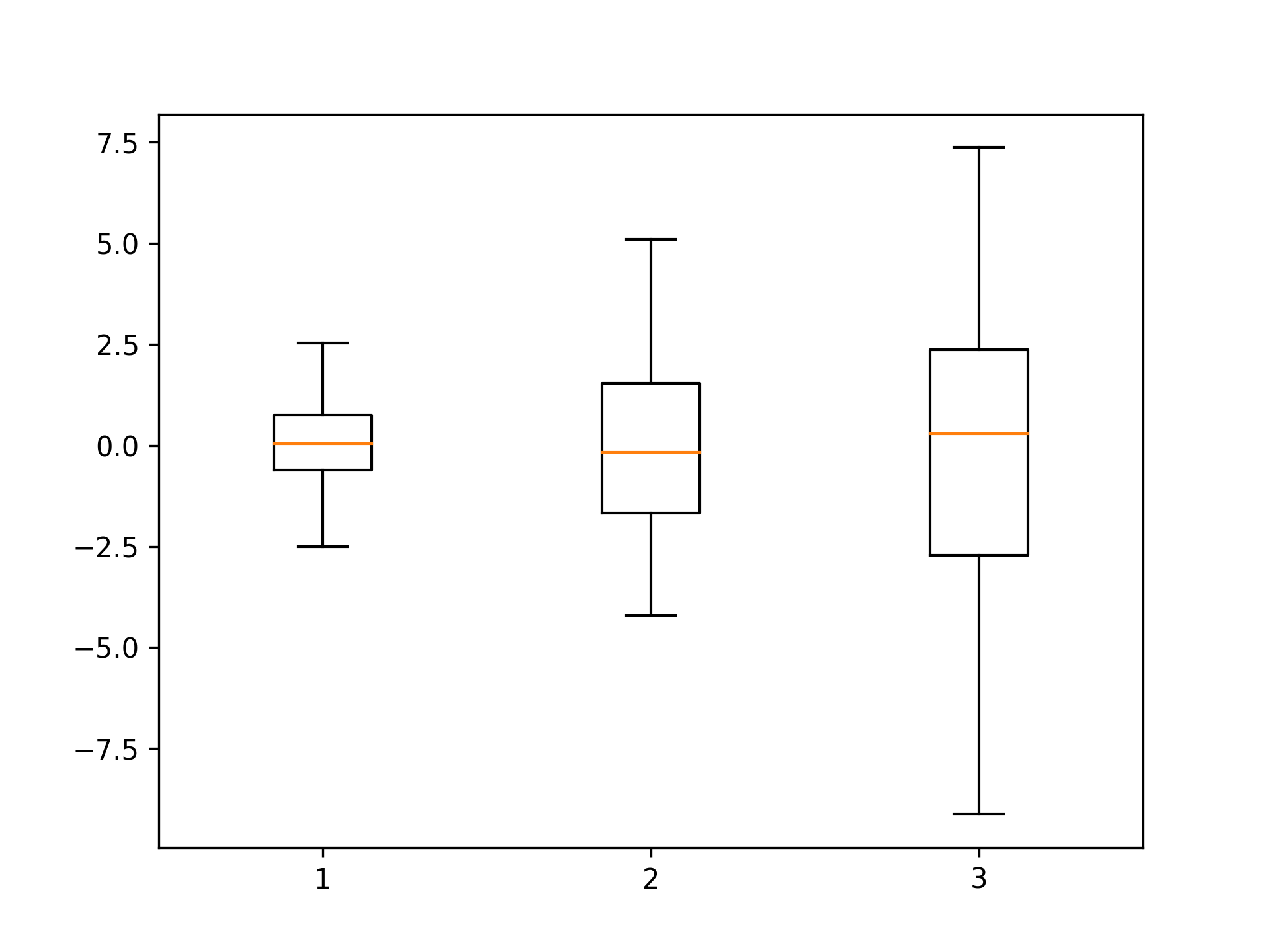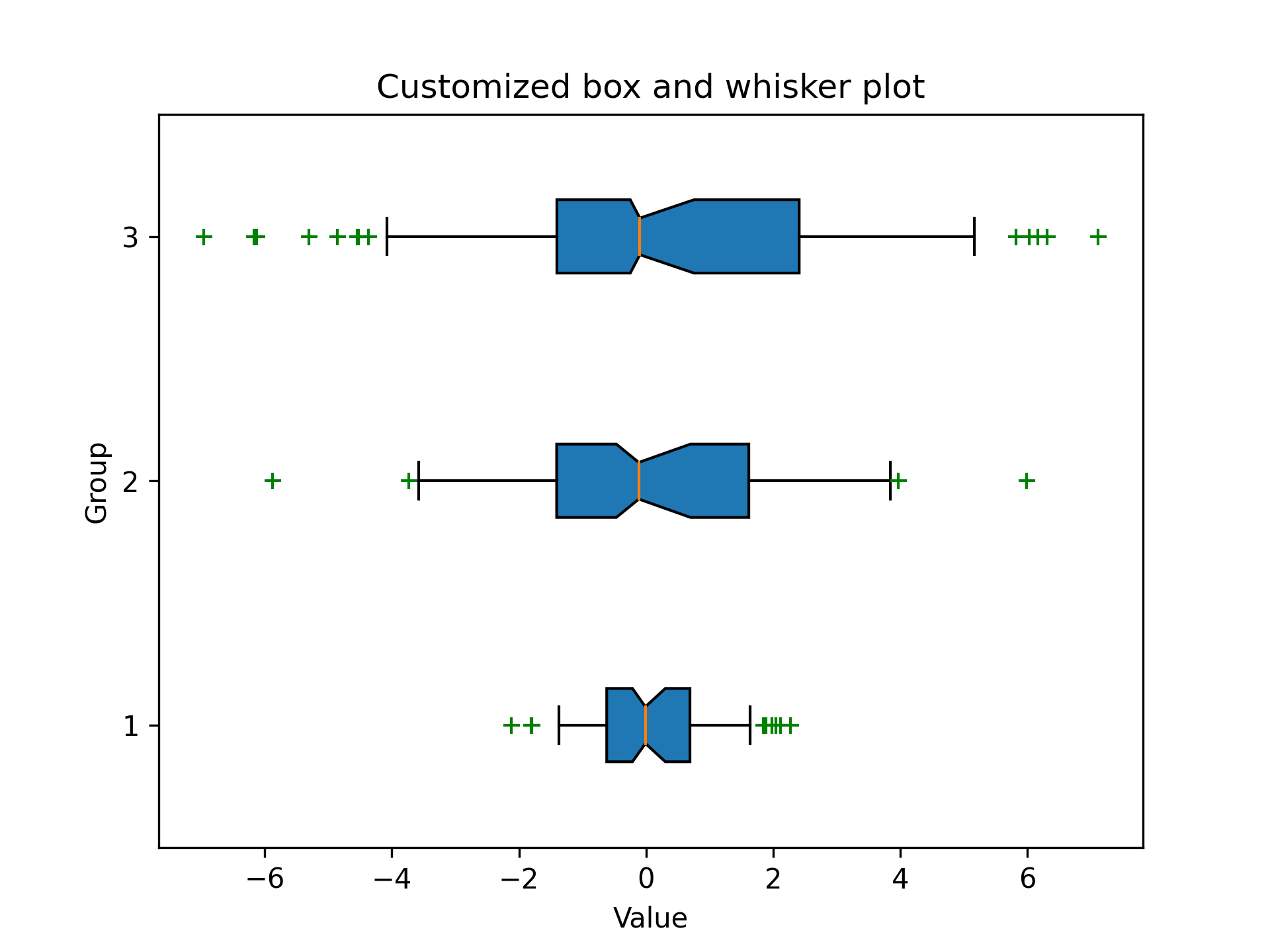# .boxplot()

The `.boxplot()` is a method in the Matplotlib library that returns a box and whisker plot based on one or more arrays of data as input.

## Syntax

``````matplotlib.pyplot.boxplot(x, notch, sym, vert, whis, bootstrap, usermedians, conf_intervals, positions, widths, patch_artist, labels, manage_ticks, autorange, meanline, zorder )
``````

The `x` parameter is required, and represents an array or a sequence of vectors. Other parameters are optional and used to modify the features of the boxplot.

`.boxplot()` takes the following arguments:

• `x` : Takes in the data to be plotted as a list, array, or a sequence of arrays. Each array represents a different dataset to be plotted in the boxplot.
• `notch`: If `True`, a notch is drawn around the median to represent the median uncertainty.
• `sym`: A parameter is used to modify the designation of outliers. By default, outliers are represented as dots, if an empty string is passed any outliers in the data will not be visible in the plot.
• `vert`: If `True`, the boxplot is drawn vertically (default). If `False`, it is drawn horizontally.
• `whis`: This parameter is used to specify the whisker length as a multiple of the IQR. The default is 1.5, which is the standard length.
• `bootstrap`: Specifies whether to bootstrap the confidence intervals around the median for notched boxplots.
• `usermedians`: This parameter is used to pass in a sequence of medians to be used for each dataset.
• `conf_intervals`: If `True`, the confidence intervals around the median are drawn as notches.
• `positions`: This parameter is used to specify the positions of the boxes in the plot.
• `widths`: This parameter is used to specify the width of the boxes.
• `patch_artist`: If `True`, the boxes will be filled with color.
• `labels`: This parameter is used to pass in a list of labels to be used for each dataset.
• `meanline`: If `True`, a line is drawn at the mean value of each dataset.
• `zorder`: This parameter is used to specify the z-order of the plot. By default, the boxplot is drawn on top of other plot elements.

## Examples

Below are the examples demonstrating the use of `.boxplot()`.

```import matplotlib.pyplot as pltimport numpy as np
# Generate some random datadata = [np.random.normal(0, std, 100) for std in range(1, 4)]
# Create a box and whisker plotplt.boxplot(data)
# Show the plotplt.show()
```

Output:```import matplotlib.pyplot as pltimport numpy as np
# Generate some random datadata = [np.random.normal(0, std, 100) for std in range(1, 4)]
# Create a box and whisker plot with some custom parametersplt.boxplot(data, notch=True, sym='g+', vert=False, whis=0.75, bootstrap=10000, usermedians=[np.mean(d) for d in data], conf_intervals=None, patch_artist=True)
# Add labels and titleplt.xlabel('Value')plt.ylabel('Group')plt.title('Customized box and whisker plot')
# Show the plotplt.show()
```

Output: